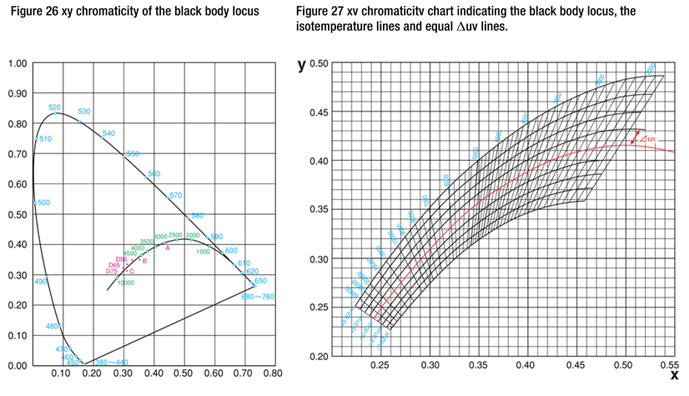## Color Measurement

An infinite number of colors surround us in our everyday lives. We all take color pretty much for granted, but it has a wide range of roles in our daily lives: not only does it influence our tastes in food and other purchases, the color of a person’s face can also tell us about that person’s health. Even though colors affect us so much and their importance continues to grow, our knowledge of color and its control is often insufficient, leading to a variety of problems in deciding product color or in business transactions involving color. Since judgement is often performed according to a person’s impression or experience, it is impossible for everyone to control color accurately using common, uniform standards. Is there a way in which we can express a given color* accurately, describe that color to another person, and have that person correctly reproduce the color we perceive? How can color communication between all fields of industry and study be performed smoothly? Clearly, we need more information and knowledge about color.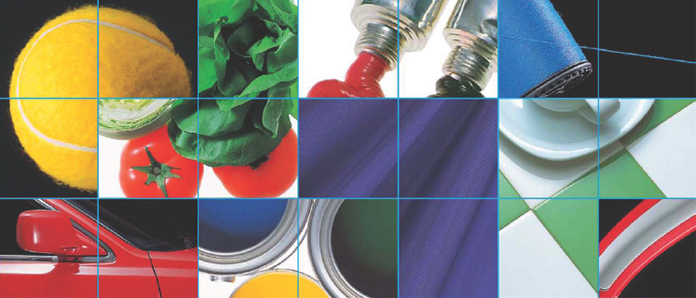2° Standard Observer and 10° Supplementary Standard Observer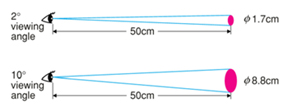The color sensitivity of the eye changes according to the angle of view (object size). The CIE originally defined the standard observer in 1931 using a 2° field of view, hence the name 2° Standard Observer. In l964, the CIE defined an additional standard observer, this time based upon a l0° field of view; this is referred to as the 10° Supplementary Standard Observer. To give an idea of what a 2° field of view is like compared to a 10° field of view, at a viewing distance of 50cm a 2° field of view would be a φ1.7cm circle while a 10° field of view at the same distance would be φ8.8cm circle. Most of the information in this booklet is based on the 2° Standard Observer. The 2° Standard Observer should be used for viewing angles of 1° to 4°; the 10° Supplementary Standard Observer should be used for viewing angles of more than 4°.

Color-Matching Functions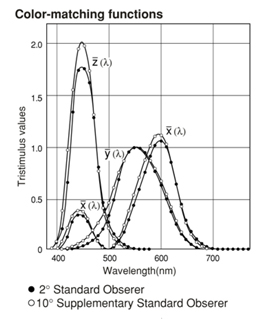The color-matching functions are the tristimulus values of the equal-energy spectrum as a function of wavelength.These functions are intended to correspond to the sensitivity of the human eye. Separate sets of three color-matching functions are specified for the 2° Standard Observer and 10° Supplementary Standard Observer.

XYZ Tristimulus Values (CIE 1931)

Tristimulus values determined based on the colormatching functions x(λ),y(λ), and z(λ) defined in 1931 by CIE; also referred to as 2° XYZ tristimulus values. They are suitable for a viewing angle of 4° or less and are defined for reflecting objects by the following formulas: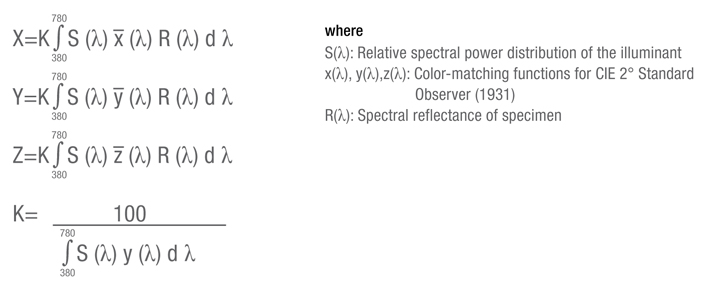XYZ Tristimulus Values (CIE 1964)

Tristimulus values determined based on the colormatching functions x10(λ), y10(λ),and z10(λ) defined in 1964 by CIE; also referred to as l0° XYZ tristimulus values. They are suitable for a viewing angle of more than 4° and are defined for reflecting objects by the following formulas: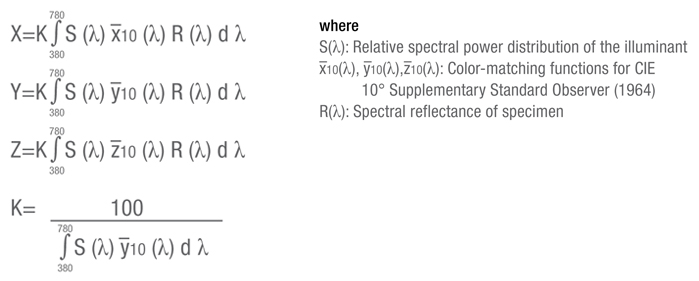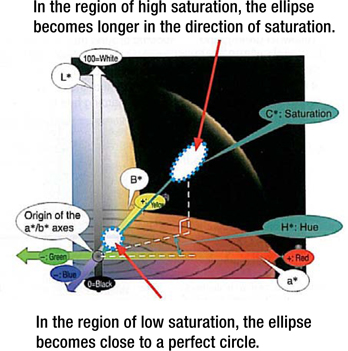Feature CIE 2000 color difference formula was developed to solve the problem of the differences in the evaluation between color meters and the human eye caused by the difference in the shape and size of the color discrimination threshold of the human eye.

The CIE 2000 color difference formula is not an attempt to build a color space in which of the color discrimination threshold of the human eye are uniform. Instead, it defines a calculation so that the color difference calculated by color meters become close to the color discrimination threshold of the human eye on the solid color space of CIE Lab (L*a*b* color space). Specifically, weigh is assigned to the lightness difference ΔL*, saturation difference ΔC*, and hue difference ΔH* by using weigh coefficients SL, SC, and Sh respectively. These weighting coefficients SL, SC, and Sh include the effects of lightness L*, saturation C*, and hue angle H. Consequently, the calculation incorporates the characteristics of the color discrimination threshold of the human eye on the color space of CIE Lab (L*a*b* color system): 1) Saturation dependency, 2) Hue dependency, and 3) Lightness dependency.

With the CIE Lab (L*a*b* color space) color difference formula, the color difference evaluation ranges represented by color difference ΔE*ab and chromaticity index difference Δa*b* were a perfect circle or a square in the L*a*b* solid color space. With CIE 2000, color difference ΔE00 is represented with an ellipse having the major axis in the direction of saturation, which is similar to the shape of the color discrimination threshold of the human eye. In the region with lower saturation, the weight coefficients SL, SC, and Sh approach 1 respectively, making the ellipse more circular.

In the region with higher saturation, the weighing coefficient SC becomes greater compared with other coefficients SL and Sh so that the ellipse becomes longer in the direction of saturation (lower sensitivity to saturation differences).

With the CIE 2000 color difference formula, the effect on the hue angle is also considered. As a result, the formula can cope with another characteristics of the color discrimination threshold of the human eye on the color space of CIE Lab (L*a*b* color space): 4) Change in the direction of the color discrimination threshold around the hue angle of 270 degrees (blue) (deviation from the direction of saturation).

XYZ Chromaticity Coordinates

The xyz chromaticity coordinates are calculated from the XYZ tristimulus values according to the following formulas: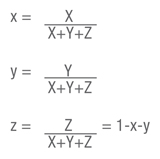If the above formulas are used with the X10 Y10 Z10 tristimulus values, the chromaticity coordinates would be x10 y10 z10.

XYZ Chromaticity Coordinates

Two-dimension diagram on which the xy or x10 y10 chromaticity coordinates can be plotted.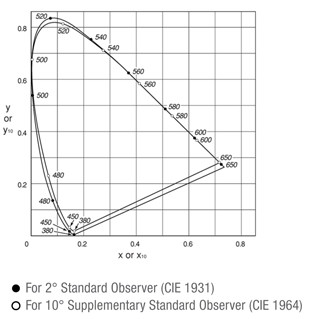L*a*b* Color Space

The L*a*b* color space (also referred to as the CIELAB space) is one of the uniform color spaces defined by the CIE in 1976. The values of L*, a*, and b* are calculated according to the formulas below: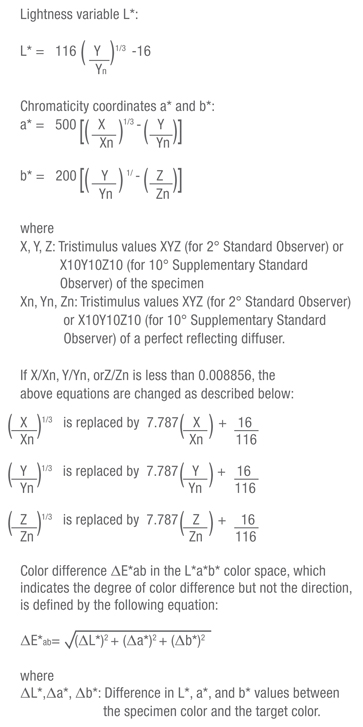L*C*h Color Space

The L*C*h color space uses the same diagram as the L*a*b* color space, but uses cylindrical coordinates. Lightness L* is the same as L* in the L*a*b* color space; Metric Chroma C* and Metric Hue-Angle h are defined by the following formulas: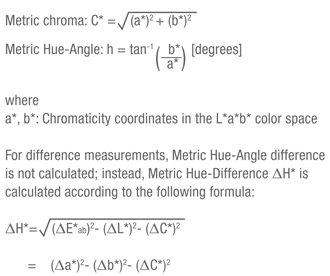The Metric Hue-Difference is positive if the Metric Hue-Angle h of the specimen is greater than that of the target and negative if the Metric Hue-Angle of the specimen is less than that of the target.

Munsell Color System

The Munsell color system consists of a series of color charts which are intended to be used for visual comparison with the specimen. Colors are defined in terms of the Munsell Hue (H; indicates hue), Munsell Value (V; indicates lightness), and Munsell Chroma (C;.indicates saturation) and written as H V/C. For example, for the color with H=5.0R, V=4.0, and C=14.0, the Munsell notation would be: 5.0R 4.0/14.0

Hunter Lab Color Space

The Hunter Lab color space was developed in 1948 by R.S. Hunter as a uniform color space which could be read directly from a photoelectric colorimeter (tristimulus method). Values in this color space are defined by the following formulas: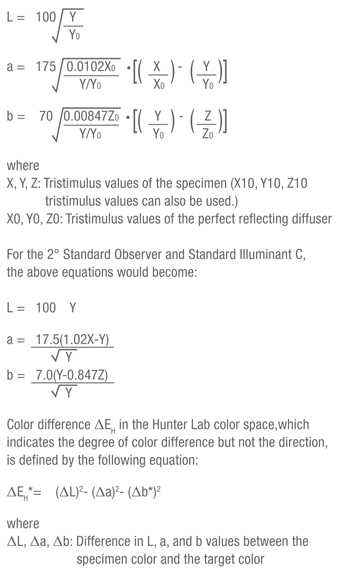Uniform Color Space

A color space in which equal distances on the coordinate diagram correspond to equal perceived color differences.

L*u*v Color Space

The L*u*v* color space (also referred to as the CIELUV space) is one of the uniform color spaces defined by the CIE in 1976. The values of L*, u*, and v* are calculated according to the formulas below: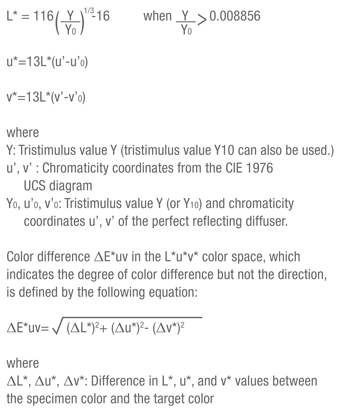CIE 1976 UCS Diagram

The CIE 1976 UCS Diagram was defined by the CIE in 1976. It is intended to provide a perceptually more uniform color spacing for colors at approximately the same luminance. The values of u’ and v’ can be calculated from the tristimulus values XYZ (or X10Y10Z10) or from the chromaticity coordinates xy according to the following formulas: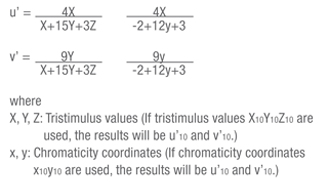CIE 1976 UCS Diagram (for 2° Standard Observer)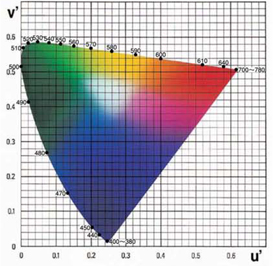ΔE*94 Color difference formula (CIE 1994)

This color difference formula modifies the lightness, saturation and hue (L*C*h*) of the L*a*b* color space by incorporating factors that correct for variation in perceived color difference magnitude in different areas of CIE 1976 (L*a*b*) Color Space. This was proposed in 1994 by the technical committee of CIE.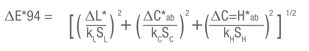Differences between object color and source color

Determining the color of an object was described previously. However, there is a difference when a color is created independently such as by a light bulb. This is called source color. The following is a simple explanation of the differences between object and source color.

Definition Differences

There are three basic factors involved when a human observes the color of an object. They are illumination, the object, and the perception of the observer. However, when a source is observed, there are only two factors: the spectral distribution of the light source and the perception of the observer. The formulas for these concepts are illustrated below.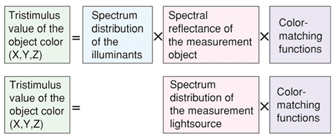For object color, it is necessary to determine and evaluate the spectral distribution of the illuminants. This is because the color appears differently when the light source varies. The illuminants are not required when the light source color ismeasured because the color of the light source itself must be determined.

Definition Differences

The geometrical conditions of the illumination and optical reception must be considered because the object color may vary under different conditions. Six types of conditions described on page 47 have been defined by the CIE. These conditions do not determine the light source color. However, there are certain angular characteristics in which hue varies depending on the type of the light source and the viewing angle, such as with LCDs. In these cases, the observation angle must be fixed at a set value.

Object Color Definition Formulas

The Hunter Lab color space was developed in 1948 by R.S. Hunter as a uniform color space which could be read directly from a photoelectric colorimeter (tristimulus method). Values in this color space are defined by the following formulas: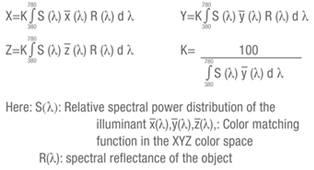Object Color Definition Formulas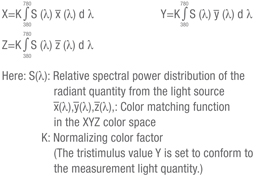Use the following equation to determine the absolute value of the measurement light quantity when S(λ)is the absolute value of the spectrum radiation density for the XYZ color space. K=683 lm/w

Definition Differences
There are several common methods to describe the light source numerically. They include the xy coordinates, the CIE 1960 UCS color intensity (u, v), the CIE 1976 UCS color intensity (u*,v*), (color temperature)*

* Refer to the page on the right for information about light source color temperature. The L*u*v* color space (CIE LUV) is also used. However, the standard light must be determined when used in the light source color because the L*u*v* color space is based on the standard color as an origin point.

As the temperature of an object increases, the emitted thermal radiation also increases. At the same time, the color changes from red through orange to white. A black body is an ideal object that absorbs all energy and emits it as radiant energy in such a manner so that its temperature is directly related to the color of the radiant energy given off. The absolute temperature of the black body is referred to as the color temperature. These colors would lie in the ideal black body locus, as indicated in the xy chromaticity chart shown in Figure 26.

Correlated color temperature is used to apply the general idea of color temperature to those colors that are close to, but not exactly, on the blackbody locus. The correlated color temperature is calculated by determining the isotemperature line on which the color of the light source is positioned. Isotemperature lines are straight lines for which all colors on the line appear visually equal; the correlated color temperature of any color on the isotemperature line is equal to the color temperature at the point where the isotemperature line intersects the blackbody locus.

The blackbody locus, the isotemperature lines and lines that indicate equal values of Δuv from the blackbody locus are illustrated in Figure 27. For example, a light source which has a color difference of 0.01 in the green direction (ΔEuv) from a black body which has a color temperature of 7000K is indicated as having a correlated color temperature of 7000K+0.0l (uv unit).

Notes
See Section IV “Color Terms” for explanation of (ΔEuv). “K” is an abbreviation for Kelvin. Kelvin is the absolute temperature scale